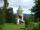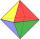Hexagonal pyramid

Base of the pyramid is a regular hexagon, which can be circumscribed in a circle with a radius of 1 meter. Calculate the volume of a pyramid 2.5 meters high.

Result

V =  2.17 m3

Solution:Leave us a comment of example and its solution (i.e. if it is still somewhat unclear...):Be the first to comment!To solve this verbal math problem are needed these knowledge from mathematics:

Tip: Our volume units converter will help you with converion of volume units. See also our trigonometric triangle calculator. Pythagorean theorem is the base for the right triangle calculator.

Next similar examples:

1. Hexagonal pyramidCalculate the volume and the surface of a regular hexagonal pyramid with a base edge length 3 cm and a height 5 cm.
2. Triangular pyramidDetermine the volume and surface area of a regular triangular pyramid having a base edge a=20 cm and a lateral edge b = 35 cm
3. 4s pyramidRegular tetrahedral pyramid has a base edge a=17 and collaterally edge length b=32. What is its height?
4. Pyramid 4sidesCalculate the volume and the surface of a regular quadrangular pyramid when the edge of the base is 4 cm long and the height of the pyramid is 7 cm.
5. Triangular pyramidCalculate the volume and surface area of a regular triangular pyramid whose height is equal to the length of the base edges 10 cm.
6. Roof 8How many liters of air are under the roof of tower which has the shape of a regular six-sided pyramid with a 3,6-meter-long bottom edge and a 2,5-meter height? Calculate the supporting columns occupy about 7% of the volume under the roof.Find the volume and surface of a regular quadrilateral pyramid if the bottom edge is 45 cm long and the pyramid height is 7 cm.
8. Pyramid heightFind the volume of a regular triangular pyramid with edge length a = 12cm and pyramid height h = 20cm.
9. 3s prismIt is given a regular perpendicular triangular prism with a height 19.0 cm and a base edge length 7.1 cm. Calculate the volume of the prism.
10. TetrahedronCalculate height and volume of a regular tetrahedron whose edge has a length 18 cm.
11. OctahedronAll walls of regular octahedron are identical equilateral triangles. ABCDEF octahedron edges have a length d = 6 cm. Calculate the surface area and volume of this octahedron.
12. Center traverseIt is true that the middle traverse bisects the triangle?
13. Theorem proveWe want to prove the sentence: If the natural number n is divisible by six, then n is divisible by three. From what assumption we started?
14. GasholderThe gasholder has spherical shape with a diameter 20 m. How many m3 can hold in?
15. SinesIn ▵ ABC, if sin(α)=0.5 and sin(β)=0.6 calculate sin(γ)The double ladder is 8.5m long. It is built so that its lower ends are 3.5 meters apart. How high does the upper end of the ladder reach?The double ladder shoulders should be 3 meters long. What height will the upper top of the ladder reach if the lower ends are 1.8 meters apart?